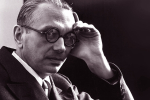# Kurt Gödel and the mechanization of mathematicsJuliette Kennedy at the TLS:

Mathematics springs from creative acts of the human imagination; yet at the same time the creativity of the mathematician is constrained by the fact of the matter. It is not up to the mathematician whether there are infinitely many prime numbers – either there are or there aren’t, and thanks to one of Euclid’s theorems, we know that there are; in fact, it is even provable.

About provability, much is known. For example, many mathematical proofs can be mechanized, that is, checked by a computer. In fact, one could imagine a completely automated practice, where one could theoretically build, say, a Turing Machine, into which one could input any mathematical conjecture and the machine would output a definite answer, yes or no, or true or false, in a finite amount of time.

One way of describing the Incompleteness Theorems (1931) of the Austrian logician Kurt Gödel is to say that he proved, in the form of a mathematical theorem, that the possibility of a fully automated mathematics can never be realized.

more here.